# RD Sharma Solutions Ex-12.2, (Part -1), Heron's Formula, Class 9, Maths Class 9 Notes | EduRev

## Class 9 : RD Sharma Solutions Ex-12.2, (Part -1), Heron's Formula, Class 9, Maths Class 9 Notes | EduRev

The document RD Sharma Solutions Ex-12.2, (Part -1), Heron's Formula, Class 9, Maths Class 9 Notes | EduRev is a part of the Class 9 Course RD Sharma Solutions for Class 9 Mathematics.
All you need of Class 9 at this link: Class 9

Q1. Find the area of the quadrilateral ABCD in which AB = 3 cm, BC = 4 cm, CD = 4 cm, DA = 5 cm and AC = 5 cm.

Solution: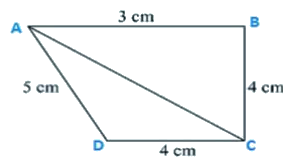For triangle ABC

AC2 = BC2+AB2

25 = 9 + 16

So, triangle ABC is a right angle triangle right angled at point R

Area of triangle ABC =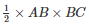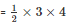= 6 cm2

From triangle CAD

Perimeter = 2s = AC + CD + DA

2s = 5 cm+ 4 cm+ 5 cm

2s   = 14 cm

s = 7 cm

By using Heron’s Formula

Area of the triangle CAD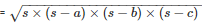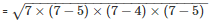= 9.16cm2

Area of ABCD = Area of ABC + Area of CAD

= (6+9.16) cm2

= 15.16cm2

Q2. The sides of a quadrilateral field, taken in order are 26 m, 27 m, 7 m, 24 m respectively. The angle contained by the last two sides is a right angle. Find its area.

Solution: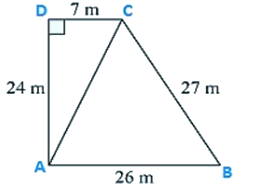Here the length of the sides of the quadrilateral is given as

AB = 26 m, BC = 27 m, CD = 7 m, DA = 24 m

Diagonal AC is joined.

Now, in triangle ADC

By applying Pythagoras theorem

AC2 = AD2+CD2

AC2 = 142+72

AC = 25 m

Now area of triangle ABC

Perimeter = 2s = AB + BC + CA

2s = 26 m + 27 m + 25 m

s = 39 m

By using Heron’s Formula

The area of a triangle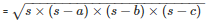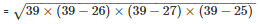= 291.84m2

Thus, the area of a triangle is 291.84m2

Now for area of triangle ADC

Perimeter = 2s = AD + CD + AC

= 25 m + 24 m + 7 m

s = 28 m

By using Heron’s Formula

The area of a triangle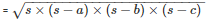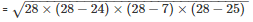= 84m2

Thus, the area of a triangle is 84m2

Therefore, Area of rectangular field ABCD

= Area of triangle ABC + Area of triangle ADC

= 291.84 + 84

= 375.8 m2

Q3. The sides of a quadrilateral, taken in order as 5 m, 12 m, 14 m, 15 m respectively, and the angle contained by first two sides is a right angle. Find its area.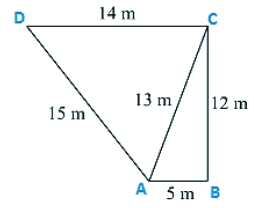Solution:

Given that the sides of the quadrilateral are

AB = 5 m, BC = 12 m, CD =14 m and DA = 15 m

Join AC

Now area of triangle ABC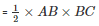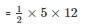= 30 m2

In triangle ABC, By applying Pythagoras theorem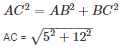AC = 13 m

Now in triangle ADC,

Perimeter = 2s = AD + DC + AC

2s = 15 m +14 m +13 m

s = 21 m

By using Heron’s Formula,

Area of the triangle PSR =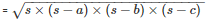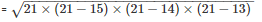= 84m2

Area of quadrilateral ABCD = Area of triangle ABC + Area of triangle ADC

= (30 + 84) m2

= 114m2

Q4. A park in the shape of a quadrilateral ABCD, has angle C = 900, AB = 9 m, BC = 12 m, CD = 5 m, AD = 8 m. How much area does it occupy?

Solution: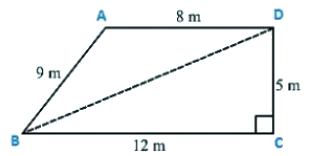Given sides of a quadrilateral are AB = 9 m, BC = 12 m, CD = 5 m, DA = 8 m.

Let us join BD

In triangle BCD , apply Pythagoras theorem

BD2 = BC2+CD2

BD2 = 122+52

BD = 13 m

Area of triangle BCD =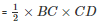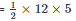= 30 m2

Now, in triangle ABD

Perimeter = 2s = 9 m + 8m + 13m

s = 15 m

By using Heron’s Formula,

Area of the triangle ABD =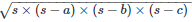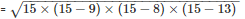= 35.49m2

Area of quadrilateral ABCD = Area of triangle ABD + Area of triangle BCD

= (35.496 + 30) m2

= 65.5m2.

Q5. Two parallel sides of a trapezium are 60 m and 77 m and the other sides are 25 m and 26 m. Find the area of the trapezium?

Solution: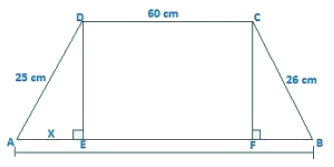Given,

Two parallel sides of trapezium are AB = 77 m and CD = 60 m

The other two parallel sides of trapezium are BC = 26 m, AD = 25m

Join AE and CF

DE is perpendicular to AB and also, CF is perpendicular to AB

Therefore, DC = EF = 60 m

Let AE = x

So, BF = 77 – 60 – x

BF = 17 – x

In triangle ADE,

By using Pythagoras theorem,

DE2 = AD2−AE2

DE2 = 252−x2

In triangle BCF,

By using Pythagoras theorem,

CF2 = BC2−BF2

CF2 = 262−(17−x)2

Here, DE = CF

So, DE2 = CF2

252−x2 = 262−(17−x)2

252−x2 = 262−(172−34x+x2)

252−x2 = 262−172+34x+x2

252 = 262−172+34x

x = 7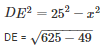DE = 24 m

Area of trapezium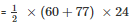Area of trapezium = 1644m2

Q6. Find the area of a rhombus whose perimeter is 80 m and one of whose diagonal is 24 m.

Solution:

Given,

Perimeter of a rhombus = 80 m

As we know,

Perimeter of a rhombus = 4×side = 4×a

4×a = 80 m

a = 20 m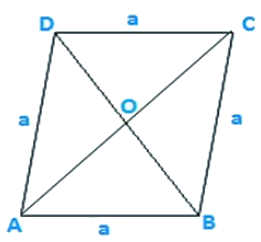Let AC = 24 m

Therefore OA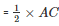OA = 12 m

In triangle AOB

OB2 = AB2−OA2

OB2 = 202−122

OB = 16 m

Also, OB = OD because diagonal of rhombus bisect each other at 90

Therefore, BD = 2 OB = 2 x 16 = 32 m

Area of rhombus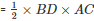Area of rhombus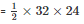Area of rhombus = 384 m2

Q7. A rhombus sheet, whose perimeter is 32 m and whose diagonal is 10 m long, is painted on both the sides at the rate of Rs 5 per meter square. Find the cost of painting.

Solution:

Given that,

Perimeter of a rhombus = 32 m

We know that,

Perimeter of a rhombus = 4×side

4×side = 32 m

4×a = 32 m

a = 8 m

Let AC = 10 m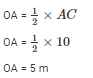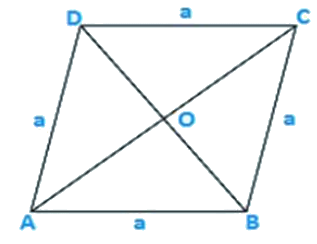By using Pythagoras theorem

OB2 = AB2–OA2

OB2 = 82–52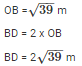Area of the sheet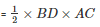Area of the sheet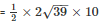Therefore, cost of printing on both sides at the rate of Rs. 5 per m2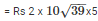= Rs. 625

Offer running on EduRev: Apply code STAYHOME200 to get INR 200 off on our premium plan EduRev Infinity!

91 docs

,

,

,

,

,

,

,

,

,

,

,

,

,

,

,

,

,

,

,

,

,

,

,

,

,

,

,

,

,

,

,

,

,

;